• 2021-05-07 22:33:50

一.克莱姆分解定理
1.概述:

克莱姆分解定理(Cramer Decomposition Theorem)是沃尔德分解定理(Wold Decomposition Theorem)的推广.沃尔德分解定理是平稳序列的理论基础,克莱姆分解定理则是非平稳序列的理论基础.该定理说明任何1个序列的波动都可视为同时受到了确定性因素和随机性因素的综合作用.产生非平稳序列的原因就在于上述2种因素中至少有1种是不稳定的

2.沃尔德分解定理

对任何1个离散平稳过程 { x t } \{x_t\} ,都可将其分解为2个不相关的平稳序列之和,其中1个为确定性序列,另1个为随机性序列,记作 x t = V t + ξ t x_t=V_t+ξ_t 其中 V t V_t 为确定性序列, ξ t = ∑ i = 0 ∞ φ i ε t − i ξ_t=\displaystyle\sum_{i=0}^\infty φ_iε_{t-i} 为随机性序列,并满足 ① φ 0 = 1 , ∑ i = 0 ∞ φ i 2 < ∞ ② { ε t } ∼ W N ( 0 , σ ε 2 ) ③ C o v ( V t , ε s ) = E ( V t , ε s ) = 0   ( t ≠ s ) ①φ_0=1,\displaystyle\sum_{i=0}^\inftyφ_i^2<\infty\\②\{ε_t\}\sim WN(0,σ_ε^2)\\③Cov(V_t,ε_s)=E(V_t,ε_s)=0\,(t\ne s)

二.差分平稳

三.ARIMA模型

四.疏系数模型

一.综述
1.概念:

"频域分析方法"(Frequency Domain Analysis)又称"(频)谱分析"(Spectral Analysis),是1种非常有用的动态数据分析方法.但其分析方法复杂,结果抽象,使用上局限性较大.其原理是:假设任何无趋势的时间序列都可以分解为若干不同频率的周期波动.其目标为:

更多相关内容
• 时间序列季节回归模型
import pandas as pd
from statsmodels.tsa.arima_model import ARMA
import matplotlib.pyplot as plt
import pmdarima as pm
import warnings
import numpy as np
from statsmodels.tsa.ar_model import AR
from sklearn.metrics import mean_squared_error as MSE
from datetime import datetime   #数据索引改为时间
import statsmodels.api as sm     #acf,pacf图
from pandas.plotting import autocorrelation_plot
from statsmodels.tsa.arima_model import ARIMA
from itertools import product                    # some useful functions
from tqdm import tqdm_notebook
from statsmodels.stats.diagnostic import acorr_ljungbox
# 过滤warning
warnings.filterwarnings('ignore')
#pmdarima==1.8.4
#numpy==1.19.5
#python3.6
def excelFile(path):
#excelFile = '降水.xlsx'
#path = 'arima_data.xlsx'
# 读取数据，指定日期列为指标，Pandas自动将“日期”列识别为Datetime格式
# data = pd.DataFrame(data,dtype=np.float64)
time_series.index = pd.to_datetime(time_series.index)
origin_timeseries = time_series
return origin_timeseries
def whiteNoiseCheck(data):
result = acorr_ljungbox(data, lags=1)
temp = result
# print('白噪声检验结果：', result)
# 如果temp小于0.05，则可以以95%的概率拒绝原假设，认为该序列为非白噪声序列；否则，为白噪声序列，认为没有分析意义
print(temp)
return temp
temp = np.array(data1)
output = pd.DataFrame(
index=['Test Statistic Value', "p-value", "Lags Used", "Number of Observations Used", "Critical Value(1%)",
"Critical Value(5%)", "Critical Value(10%)"], columns=['value'])
output['value']['Test Statistic Value'] = t
output['value']['p-value'] = t
output['value']['Lags Used'] = t
output['value']['Number of Observations Used'] = t
output['value']['Critical Value(1%)'] = t['1%']
output['value']['Critical Value(5%)'] = t['5%']
output['value']['Critical Value(10%)'] = t['10%']
return t,t['1%']
def stableCheck(timeseries):
# 可视化移动12期的均值和方差,未改动,没用到
rol_mean = timeseries.rolling(window=12).mean()
rol_std = timeseries.rolling(window=12).std()
# 绘图
fig = plt.figure(figsize=(12, 8))
orig = plt.plot(timeseries, color='blue', label='Original')
mean = plt.plot(rol_mean, color='red', label='Rolling Mean')
std = plt.plot(rol_std, color='black', label='Rolling Std')
plt.legend(loc='best')
plt.title('Rolling Mean & Standard Deviation')
plt.show()
print('Results of Dickey-Fuller Test:')
# 对检验结果进行语义描述
dfoutput = pd.Series(dftest[0:4], index=['Test Statistic', 'p-value', '#Lags Used', 'Number of Observations Used'])
for key, value in dftest.items():
dfoutput['Critical Value (%s)' % key] = value
print(dfoutput)
def season_fiff(data,n):
time_series_diff1 = data.diff(1).dropna()
# 在一阶差分基础上进行季节性差分差分
time_series_diff2 = time_series_diff1.diff(n).dropna()
#stableCheck_result2 = stableCheck(time_series_diff2)
return time_series_diff2
def parameter_generation(len_season):
ps = range(2, 5)
d = 1  # 做了一次一阶差分
qs = range(2, 5)
Ps = range(0, 2)
D = 1  # 季节性差分阶数。
Qs = range(0, 2)
s = len_season  # season length is still 24
# creating list with all the possible combinations of parameters
parameters = product(ps, qs, Ps, Qs)
parameters_list = list(parameters)
return parameters_list

def optimizeSARIMA(parameters_list, d, D, s, data1):
results = []
best_aic = float("inf")

for param in tqdm_notebook(parameters_list):
# we need try-except because on some combinations model fails to converge
try:
model = sm.tsa.statespace.SARIMAX(data1, order=(param, d, param),
seasonal_order=(param, D, param, s)).fit(disp=-1)
except:
continue
aic = model.aic
# saving best model, AIC and parameters
if aic < best_aic:
best_model = model
best_aic = aic
best_param = param
results.append([param, model.aic])

result_table = pd.DataFrame(results)
result_table.columns = ['parameters', 'aic']
# sorting in ascending order, the lower AIC is - the better
result_table = result_table.sort_values(by='aic', ascending=True).reset_index(drop=True)
return result_table

def model_struct(parameters_list,data1,d,D,s):

result_table = optimizeSARIMA(parameters_list, d, D, s, data1)
p, q, P, Q = result_table.parameters
best_model = sm.tsa.statespace.SARIMAX(data1, order=(p, d, q),
seasonal_order=(P, D, Q, s)).fit(disp=-1)
#print(best_model.summary())
return best_model

def mean_absolute_percentage_error(y_true, y_pred):
return np.mean(np.abs((y_true - y_pred) / y_true)) * 100

def plotSARIMA(series, model, n_steps,d,s):

data = series.copy()
data.columns = ['actual']
data['sarima_model'] = model.fittedvalues
# making a shift on s+d steps, because these values were unobserved by the model
# due to the differentiating
data['sarima_model'][:s + d] = np.NaN

# forecasting on n_steps forward
forecast = model.predict(start=data.shape, end=data.shape + n_steps)
forecast = data.sarima_model.append(forecast)
# calculate error, again having shifted on s+d steps from the beginning
error = mean_absolute_percentage_error(data['actual'][s + d:], data['sarima_model'][s + d:])
print(error)
date = forecast.index[data.shape:]
pre_y = forecast.values[data.shape:]
output_x = [x.strftime('%F') for x in date]
output_y = pre_y.tolist()
return output_x,output_y

def model_struct_auto(time_series,parser_m):
# 将数据分为训练集data_train和测试集data_test 。
split_point = int(len(time_series) * 0.85)
# 确定训练集/测试集
data_train, data_test = time_series[0:split_point], time_series[split_point:len(time_series)]
# 使用训练集的数据来拟合模型
built_arimamodel = pm.auto_arima(data_train, start_p=0,  # p最小值
start_q=0,  # q最小值
max_p=5,  # p最大值
max_q=5,  # q最大值
m=parser_m,  # 季节性周期长度，当m=1时则不考虑季节性
d=None,  # 通过函数来计算d
seasonal=True, start_P=0, D=1, trace=True,
error_action='ignore', suppress_warnings=True,
stepwise=False)  # stepwise为False则不进行完全组合遍历
print(built_arimamodel.summary())
#可视化
#built_arimamodel.plot_diagnostics()
#plt.show()

if __name__ == '__main__':
time_series=excelFile('降水.xlsx')
s=12
d = 1
D = 1
data1=time_series.y
if t1>t2:
data=season_fiff(time_series, s)
t=whiteNoiseCheck(data)
if t<0.05:
parameters_list=parameter_generation(s)
best_model=model_struct(parameters_list,data1,d,D,s)
x,y=plotSARIMA(time_series, best_model, 20,d,s)#预测20个
print(x)
print(y)

#pmdarima==1.8.4
#numpy==1.19.5
#python3.6
#代码有借鉴，有修改


运行结果数据集
链接：https://pan.baidu.com/s/1SKZwtLgh3tWBePKTJjFCkw
提取码：4kwb
emmmmm，有问题的话请指出，我也是小白

展开全文回归 python 机器学习
• 并应用熵权法确定各参数权重，建立工作面涌水量预测的非线性回归修正模型，并将模拟预测结果与忽略季节效应的ARIMA模型预测的涌水量进行对比，结果表明，建立的非线性时间序列模型计算的涌水量更为接近实测涌水量，...
• 基于时间序列的加法季节模型和乘法季节模型以及两者之间的比较如下： 实验数据来源与1962年到1991年德国工人季度失业率序列： 一、加法季节模型 1、首先加载所需的包： 2、导入数据，并将数据转换为季节...

基于时间序列的加法季节模型和乘法季节模型以及两者之间的比较如下：

实验数据来源与1962年到1991年德国工人季度失业率序列：

一、加法季节模型

1、首先加载所需的包：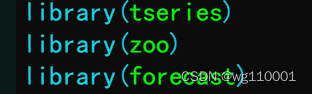2、导入数据，并将数据转换为季节数据：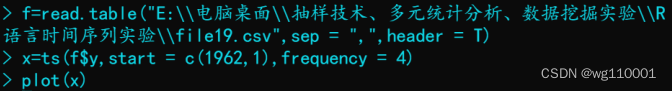3、得到时序图如下，发现既有季节效应又有上升趋势效应：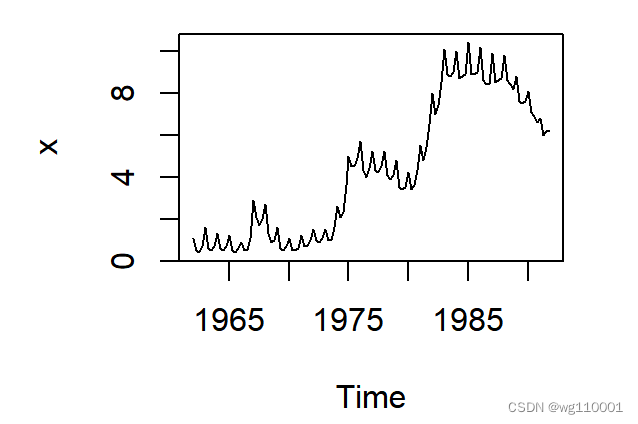4、接着我们对数据进行一阶四部差分：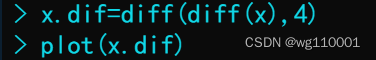一阶四步差分时序图如下：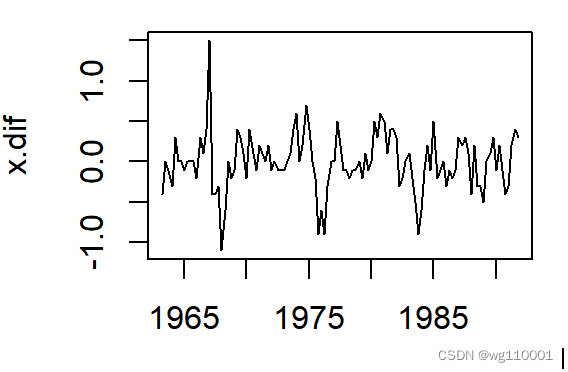从一阶四步差分时序图可以看出，我们消除了上升趋势效应以及季节效应，即为平稳序列。

5、接着画出差分后序列相关图和偏自相关图：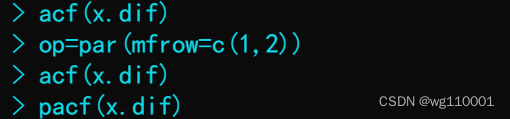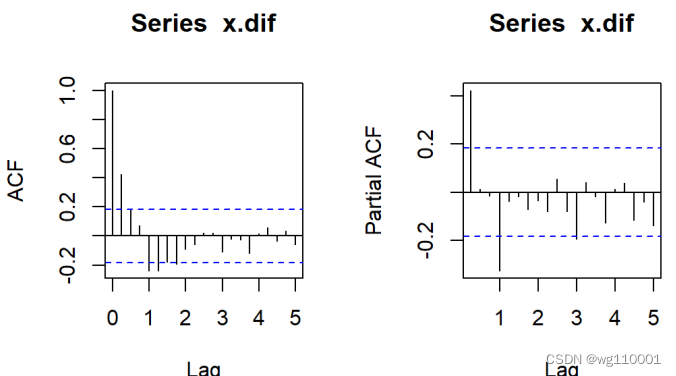结果显示自相关具有拖尾性，偏自相关图在一阶和3阶之后都落入两倍的标准差之内了，综合考虑，最后拟合一个假发季节模型:ARIMA((1,4),(1,4),0))

6、模型拟合结果及优化结果对比显示：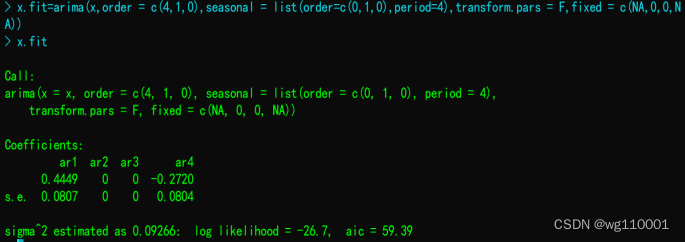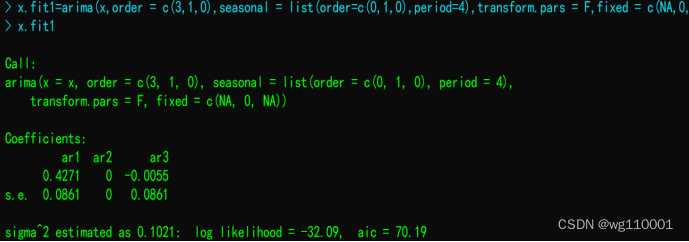结果显示ARIMA((1,4),(1,4),0))模型aic数值较小，ARIMA((1,4),(1,4),0))模型较好。

7、运用forecast函数进行预测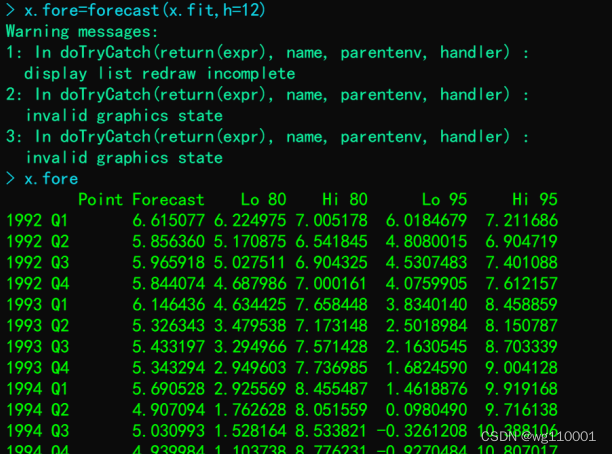8、预测结果可视化：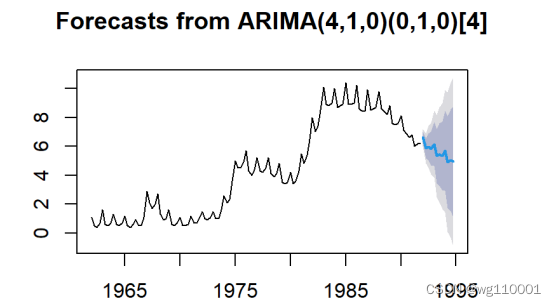9、模型的残差序列白噪声检验：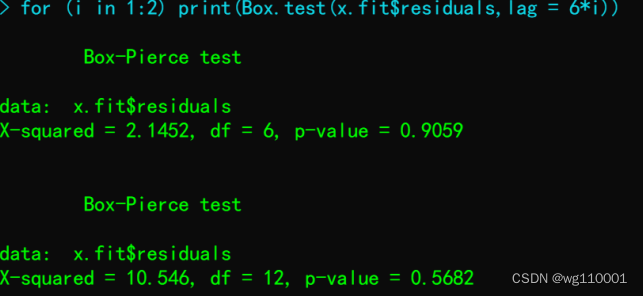p值较大，说明加法季节模型很好的提取了数据信息，模型拟合效果很理想。

二、乘法季节模型

数据来源于1948年到1981年美国女性月度事业率序列

1、读取数据，并将数据转换为时间序列数据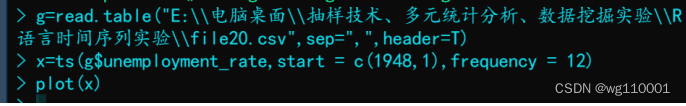2、输出时间序列时序图，发现既有周期性又有上升趋势效应：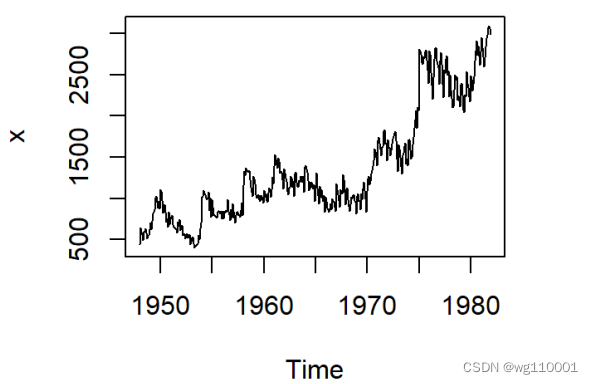接着我们对数据进行一阶12步差分：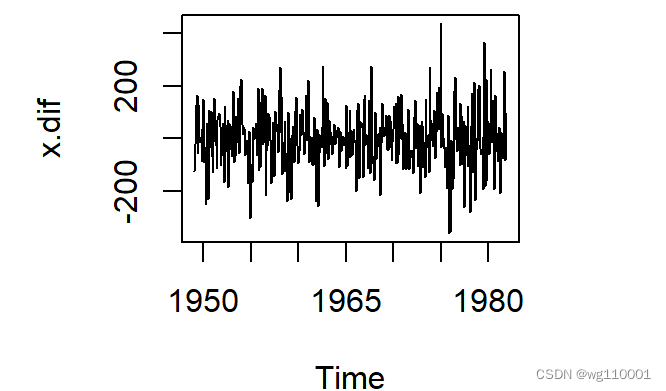从一阶12步差分时序图可以看出，我们消除了上升趋势效应以及周期效应，即为平稳序列。

3、接着画出差分后序列相关图和偏自相关图：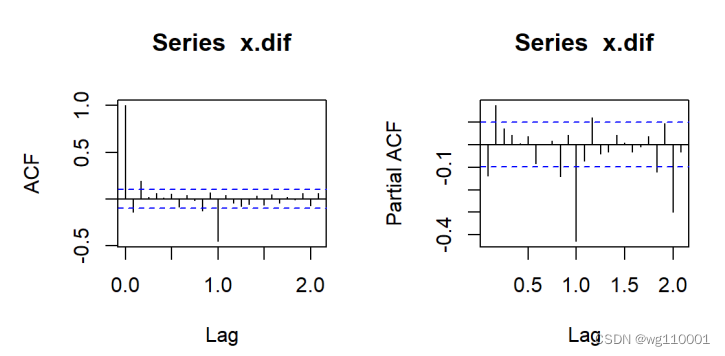结果显示自相关和偏自相关都具有拖尾性。

4、首先拟合一个加法季节模型:ARIMA(1,12),1)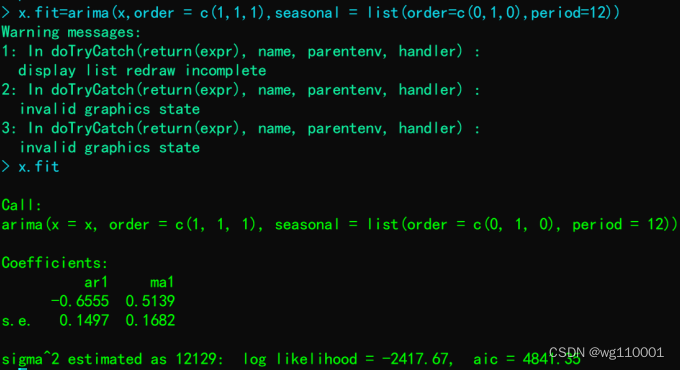5、模型残差序列白噪声检验：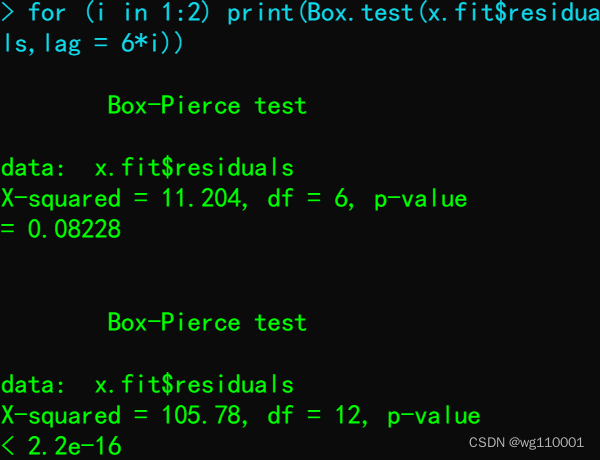由结果可得6阶时p值为0.08228，12阶时p值为2.2e-16,取α值为0.1时，均拒绝原假设，说明残差为非白噪声序列，说明时间序列选择加法季节效应模型不正确。

6、接着我们选择乘积季节效应进行拟合，关键在于阶数的选择，结束的选择，我们仍选择用自相关和偏自相关图来观察：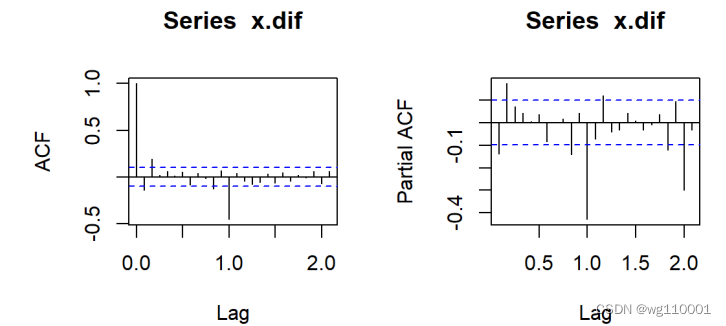从短期来看，自相关在短期和偏自相关都是拖尾的，自相关认为时1阶即一个周期截尾的，偏自相关系数1阶拖尾，p取0，q取1。

7、6期乘法季节序列模型预测：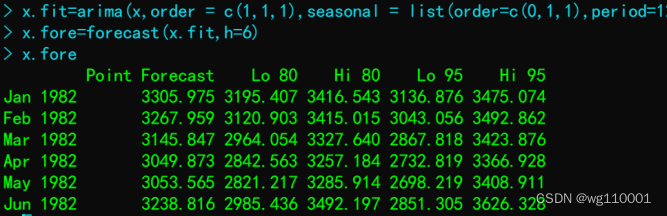8、结果可视化：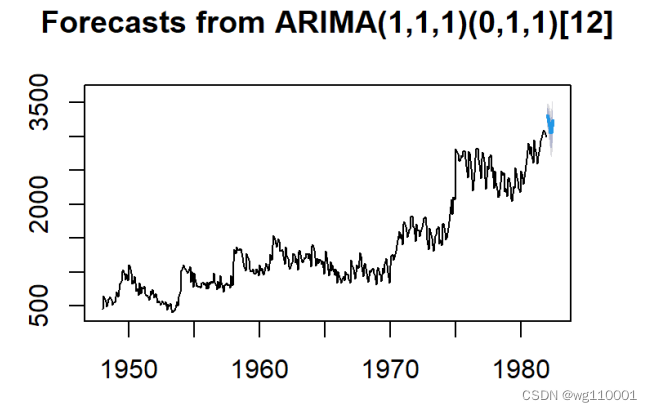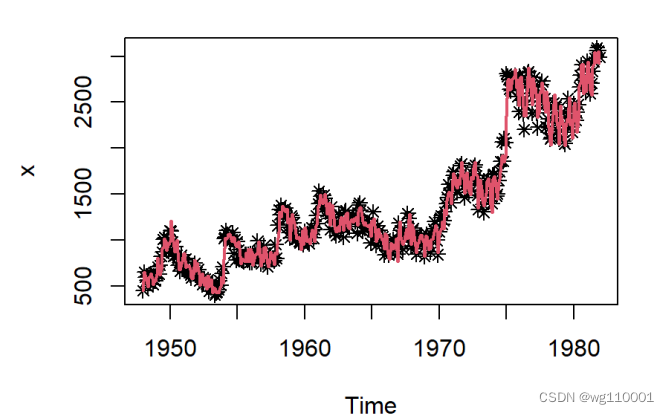9、模型残差序列白噪声检验：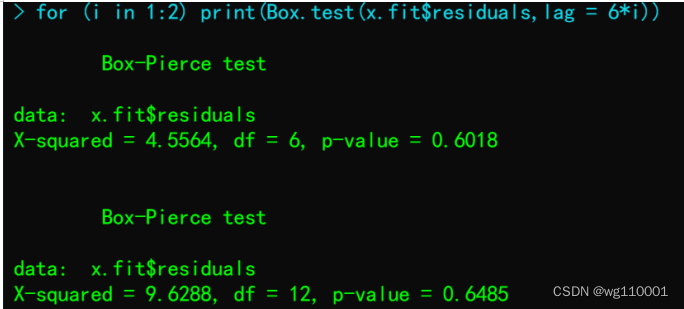p值较大，说明乘法季节模型很好的提取了数据信息，模型拟合效果很理想。

展开全文机器学习 人工智能 数据挖掘
• 实验 名称 疏系数模型季节模型 实验 ... 1、简单季节模型 ... 1、掌握疏系数模型 ... 2、熟练建立季节模型 ...简单季节模型结构 ... SPSS建立时间序列乘法季节模型实战案例 Pyth...
 实验 名称 疏系数模型 和季节模型 实验 内容 1、简单季节模型 实验 目的 1、掌握疏系数模型 2、熟练建立季节模型

目录

简单季节模型结构

模型建立

时序图

差分平稳化

白噪声检验

模型定阶

参数估计和模型检验

模型预测

推荐阅读

# 简单季节模型结构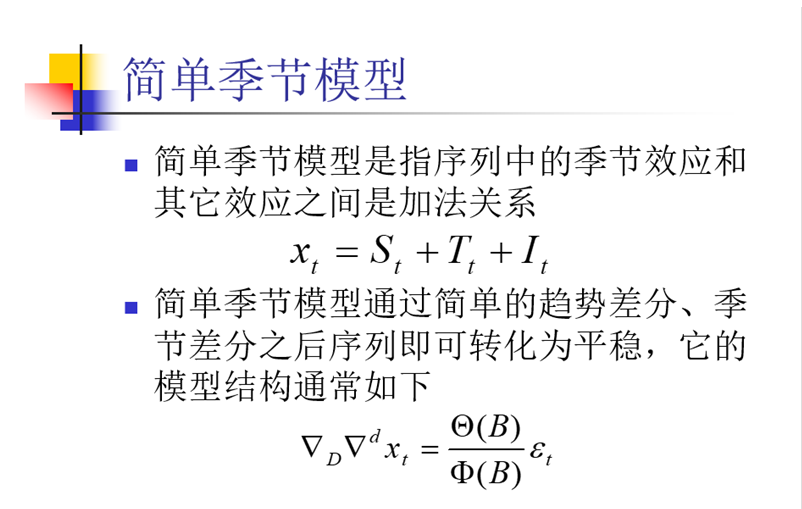# 模型建立

## 时序图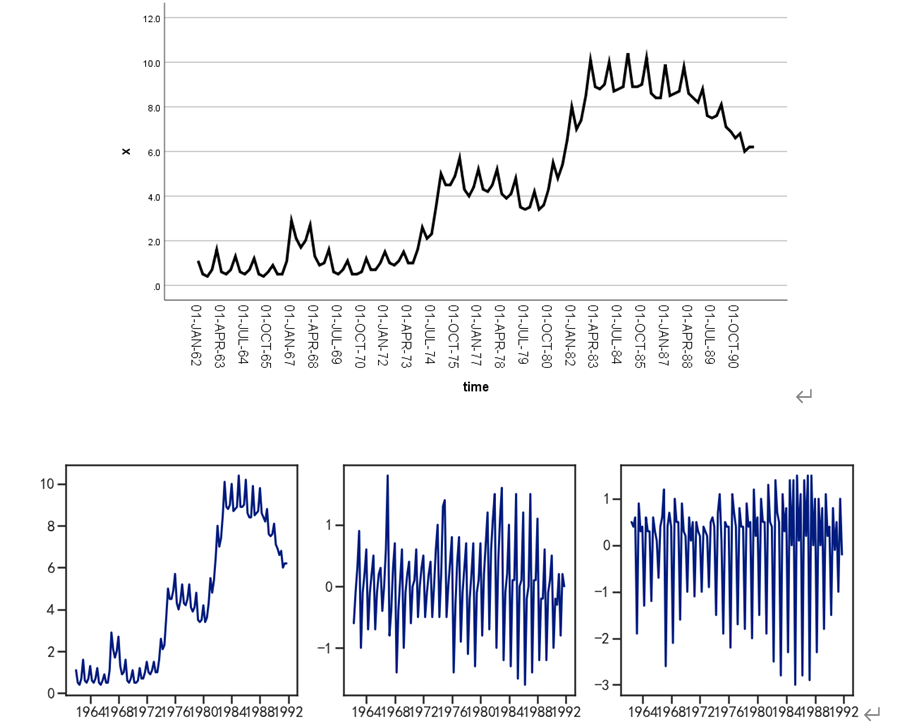时序图显示，该序列既包含长期趋势又包含以年为周期的季节效应

## 差分平稳化

对原序列做1阶差分消去趋势，再做4步差分消去季节效应的影响，差分后序列时的时序图：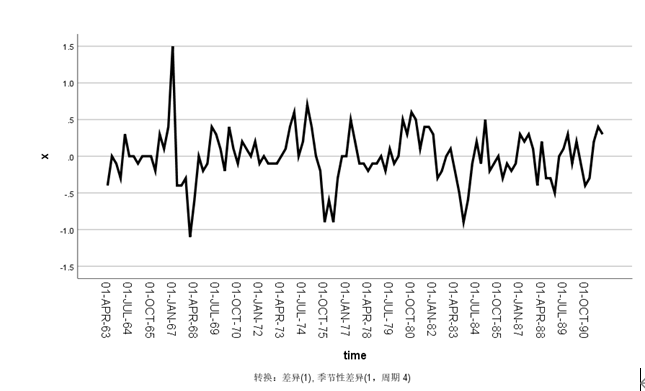单位根检验：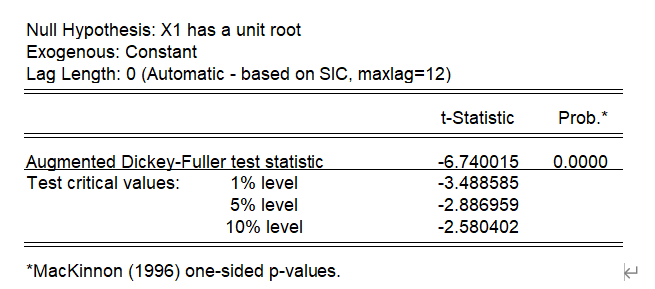## 白噪声检验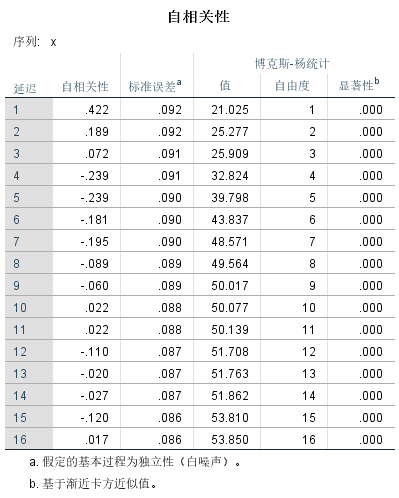检验结果显示，差分后序列时平稳非白噪声序列，需要对差分后的序列进行进一步拟合ARMA模型。

## 模型定阶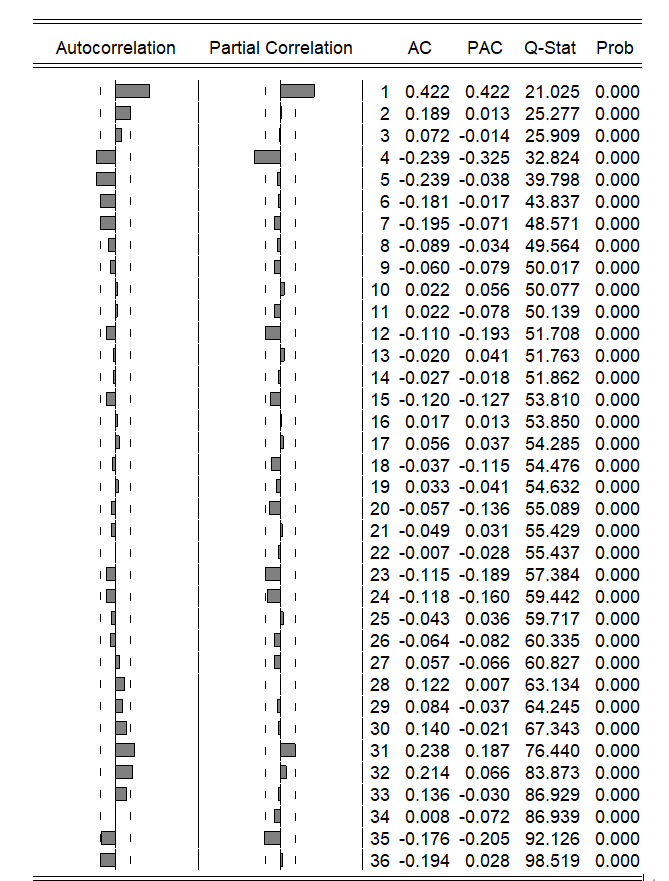自相关图显示出明显的下滑轨迹，这是典型的拖尾属性。偏自相关图除了1阶和4阶偏自相关系数显著大于2倍标准差。所以尝试拟合ARIMA(4,1,0)*(0,1,0)4

## 参数估计和模型检验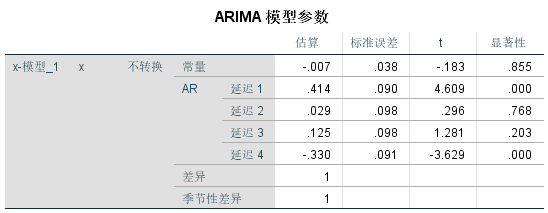x2,x3，P>α，不通过显著性检验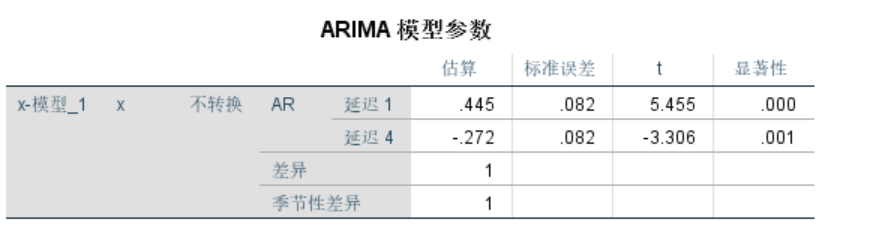模型的显著性检验：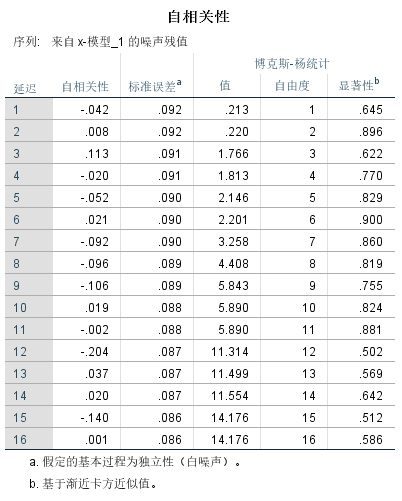检验结果显示，残差序列为白噪声序列，参数显著性检验显示两个参数均显著非0。

## 模型预测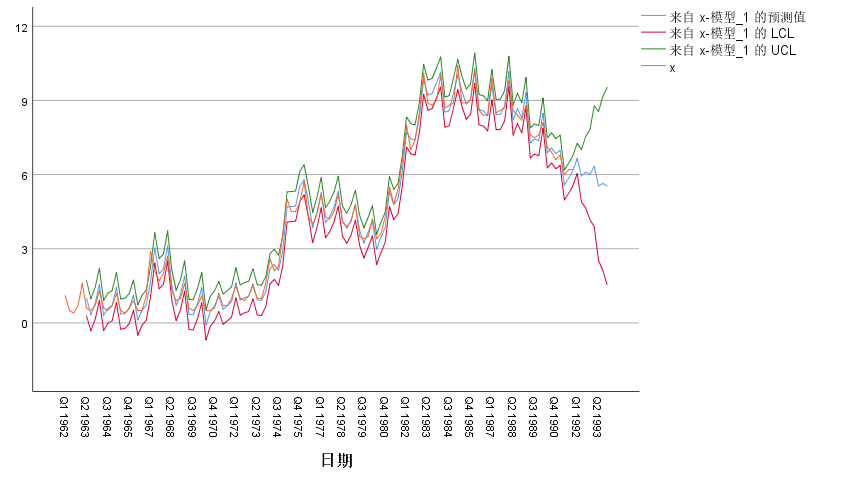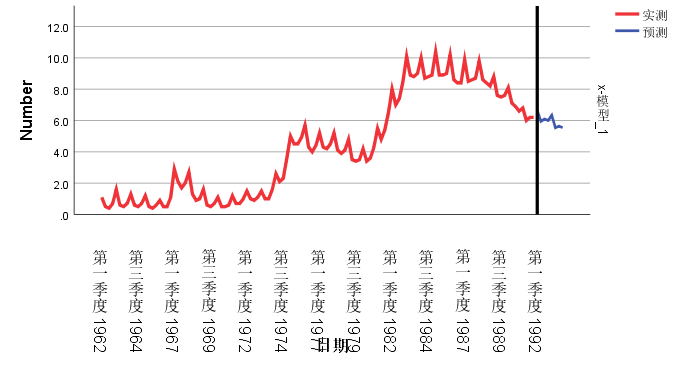> 到这里就结束了，如果对你有帮助你，欢迎点赞关注，你的点赞对我很重要

展开全文• 时间序列分析-------乘法季节模型 实验 名称 乘法季节模型 实验 内容 乘法季节模型 实验 目的 2、熟练建立乘法季节模型 乘法季节...
• 如果想判断季节性，最初只需要先去做AR、MA等模型的相关测试，例如先去计算PACF，看收敛情况，选择AR、MA等模型，然后选择好，比如说一阶测试的话，就要先去OLS回归，并且取残差，看残差是否有Autocorrelation，就看...回归
• 前言：前一篇介绍了对平稳时间序列的分析方法和流程，在没有考虑季节效应的情况下，模型建立的并不成功。本篇以美国1980年-2015年月度失业率为对象，进行一个更为完善的、有季节效应的非平稳时间序列分析流程。 理论...
• 对于季节时间序列的预测，可以采用“季节系数法”来预测时间序列的变化趋势。在时间序列问题中，季节并不单纯代表一年四季，一些既有季度性、周期性的时间序列也可以用季节系数法来进行预测，例如月份。其步骤如下...
• 时间序列季节性分解 可将一个序列分解成一个季节性成分、一个组合趋势和循环成分、一个“误差”成分。 时间序列分两种模型：乘法模型和加法模型，对上面3个分量做乘法或加法。 如果季节性波动的幅度不随着序列水平...
• 时间序列分析大致可以分成三部分，分别是描述过去，分析规律和预测未来，本次讲将主要介绍时间序列分析中常用的三种模型季节分解、指数平滑方法和ARIMA模型，并将结合SPSS软件对时间序列数据进行建模。...ARIMA
• ARMA是一种平稳时间序列模型，即均值和协方差不随时间的平移而改变。 ARMA有三种类型 AR序列 MA序列 ARMA序列 但是由于ARMA只能处理平稳序列，而现实中的问题往往有趋势性或周期性等。为了得到平稳序列，我们对...matlab ARIMA
• 6.1 因素分解理论 6.2因素分解模型 6.2.1因素分解模型的选择 6.2.2趋势效应的提取 简单中心移动平均的良好属性 ...6.2.3 季节效应的提取 6.2.4 X11季节调节模型 X11模型分析步骤： ​确定性因素分解函数： 小结
• 时间序列数据的频率高于季度或月度时，许多预测程序在分析季节性影响方面遇到了障碍。 澳大利亚蒙纳士大学的研究人员在美国统计协会杂志（JASA）上发表了一篇有趣的论文，以及一个R程序，以处理这种情况 - 可称为...R语言
• 时间序列的分析的步骤是先对数据进行平稳性和非白噪声检验（如不满足需对数据进行平滑或差分等预处理），然后才是模型调参跟预测，因此本文分为2大部分介绍，答题思路见脑图。 一、数据准备&探索 1、平稳性 ...python
• 时间序列模型 - Prophet1.时间序列简介1.1 时间序列 - 平稳性检验1.1.1 log法1.1.2 差分法1.2 平稳性的单位根检验2.ARIMA3.Prophet3.1 Prophet的优点3.2 安装Prophet3.3 数据处理3.4 预测4.LSTM4.1 数据处理4.2 训练...python
• 序列分解1、非季节时间序列分解 移动平均MA(Moving Average)①SAM（Simple Moving Average） 简单移动平均，将时间序列上前n个数值做简单的算术平均。 SMAn=(x1+x2+…xn)/n②WMA(Weighted Moving Average) ...R
• end yt dyt yhat' % 预测值 具有季节性特点的时间序列预测 季节可以指自然季节，也可以指销售季节等等。例如羽绒服肯定是冬季销量夏季的。下面介绍一种季节系数法，计算步骤如下： 例题： clc,clear format long g ...数据挖掘 matlab
• 现在我们研究的是时间序列模型。 分类 与普通的数值拟合不同，时间序列的拟合需要考虑多种因素，本质上是一种高级的拟和方式。同时也是一种黑箱模型， 小结一下：多参数对多/单结果：神经网络模型 单/复参数-单结果...数据建模
• 时间序列是一种特殊类型的数据集，其中随时间测量一个或多个变量。机器学习 深度学习 人工智能
• SPSS(十九)SPSS之时间序列模型（图文+数据集） 时间序列是指将同一统计指标的数值按其发生的时间先后顺序排列而成的数列。正如人们常说，人生的出场顺序很重要，时间序列中隐藏着一些过去与未来的关系。时间序列...自相关偏自相关
• 时间序列在量化投资中具有广泛的应用，时间序列分析是金融量化分析重要组成部分，是构建策略时需要重点考虑的问题。一般来讲，时间序列的分析技术在量化投资中的应用有： 对未来的资产价格预测 模拟序列 ...
• ## 时间序列模型Prophet使用详细讲解

万次阅读 多人点赞 2018-10-26 20:39:34
默认情况下，将展示趋势、时间序列的年度季节性和周季节性。如果之前包含了节假日，也会展示出来。 # 预测的成分分析绘图，展示预测中的趋势、周效应和年度效应 m.plot_components(forecast); 注： 一个...Prophet
• 来源：机器学习研习院大家好，我是阳哥！时间序列预测是机器学习中一个经常被忽视的重要领域。时间序列在观察之间添加了显式的顺序依赖性：时间维度。这个额外的维度既是一个约束，也是一个提供额外信息...python 机器学习 人工智能 深度学习
• 文章目录1 Causal Impact与贝叶斯结构时间序列模型1.1 观测数据下Causal Impact的背景由来1.2 贝叶斯结构时间序列模型1.3 谷歌的Causal Impact2 一些案例2.1 CausalImpact的时序选择2.2 日文案例:CausalImpactの理解...人工智能 机器学习 数据挖掘
• 时间序列模型：严格来说包含4个要素，Trend/趋势、Circle/循环、Seasonal /季节性和不规则要素。但是实际中C和S貌似更多的归为一个。 一组时间序列数据有可能包含T和S，这都导致数据集不平稳。因为T会造成均值跟着...python 差分法
• 数据来自澳大利亚莫纳什大学统计学教授Rob Hyndman创建的时间序列数据库（TSDB），数据库涵盖近800个时间序列数据（经济、水文、气象、农业等）； 网址：http://datamarket.com/data/list/?q=provider:tsdl 数据集...
• 目录1 概述2 时间序列中的季节成分3 机器学习的好处4 季节性的类型4 消除季节性5 每日最低温度数据6 差分6.1 每日数据的差分6.2 月平均数据的差分7 通过建模来进行修正 1 概述 时间序列数据可能包含一些季节性的...python 机器学习
• 上篇说明了分解非季节性数据的方法。就是通过TTS包的SMA（）函数进行简单移动平均平滑。让看似没有规律或没有趋势的曲线变的有规律或趋势。然后再进行时间序列曲线的...对于可以使用相加模型进行描述的时间序列中...
•机器学习 人工智能 深度学习 回归 算法...